U-Substitution

### THE METHOD OF U-SUBSTITUTION

The following problems involve the method of u-substitution. It is a method for finding antiderivatives. We will assume knowledge of the following well-known, basic indefinite integral formulas :
1.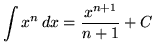2.3.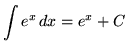4.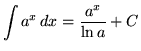, where a is a constant
5.6.7.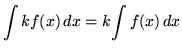, where k is a constant
8.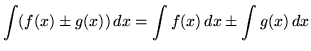The method of u-substitution is a method for algebraically simplifying the form of a function so that its antiderivative can be easily recognized. This method is intimately related to the chain rule for differentiation. For example, since the derivative of ex is,

it follows easily that.

However, it may not be obvious to some how to integrate.

Note that the derivative of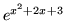can be computed using the chain rule and is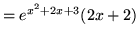.

Thus, it follows easily that.

This is an illustration of the chain rule "backwards". Now the method of u-substitution will be illustrated on this same example. Begin with,

and let

u = x2+2x+3 .

Then the derivative of u is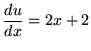.

Now "pretend" that the differentiation notation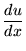is an arithmetic fraction, and multiply both sides of the previous equation by dx gettingor

du = (2x+2) dx .

Make substitutions into the original problem, removing all forms of x , resulting in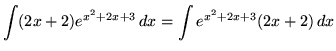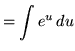= e u + C

= e x2+2x+3 + C .

Of course, it is the same answer that we got before, using the chain rule "backwards". In essence, the method of u-substitution is a way to recognize the antiderivative of a chain rule derivative. Here is another illustraion of u-substitution. Consider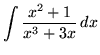.

Let

u = x3+3x .

Then (Go directly to the du part.)

du = (3x2+3) dx = 3(x2+1) dx ,

so that

(1/3) du = (x2+1) dx .

Make substitutions into the original problem, removing all forms of x , resulting in.

Most of the following problems are average. A few are challenging. Make careful and precise use of the differential notation dx and du and be careful when arithmetically and algebraically simplifying expressions.

• PROBLEM 1 : Integrate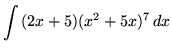.

• PROBLEM 2 : Integrate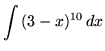.

• PROBLEM 3 : Integrate.

• PROBLEM 4 : Integrate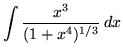.

• PROBLEM 5 : Integrate.

• PROBLEM 6 : Integrate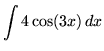.

• PROBLEM 7 : Integrate.

• PROBLEM 8 : Integrate.

• PROBLEM 9 : Integrate.

• PROBLEM 10 : Integrate.

• PROBLEM 11 : Integrate.

• PROBLEM 12 : Integrate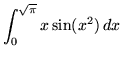.

The following problems require u-substitution with a variation. I call this variation a "back substitution". For example, if u = x+1 , then x=u-1 is what I refer to as a "back substitution".

• PROBLEM 13 : Integrate.

• PROBLEM 14 : Integrate.

• PROBLEM 15 :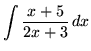.

• PROBLEM 16 :.

• PROBLEM 17 :.

• PROBLEM 18 : Integrate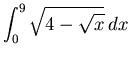.